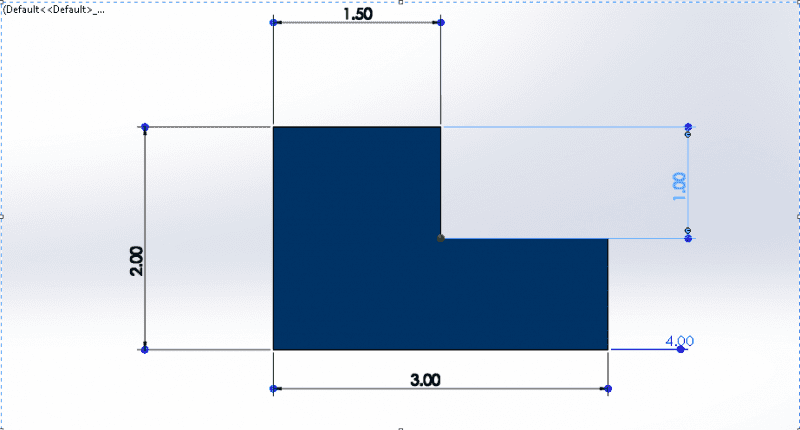# Calulate rapid prototyping stl build time

I am not sure where this goes , if this is the wrong place sorry.

Time required to build the part.The 4 is the total length of the part.

It also says the scanning speed is 80ft/min
the spot size is 0.010 in
delay time is 7 sec/layer

The formula that is given

Ti=(Ai/(vD))+Td

Where
Ti= time to complete layer
Ai=area of the layer
v= average scanning speed of the laser beam at the surface
D= diameter of the spot size
Td= delay time between layers to re position the worktable

I want to know the steps of this forumla.

billy_joule
Are you familiar with how a 3D printer works?
If you put units into the formula and it should become clear.

Ti=(Ai/(vD))+Td
Time taken = (area to be covered / (speed of print head * width of printed line) + time to reposition for next layer
Seconds = m^2 / (m/s * m) + Seconds

If you know the layer thickness you can expand the formula to give a total time to make the part.

Of course most parts are not printed with 100% infill so actual time may be significantly shorter.
The actual time is difficult to calculate accurately (by hand) as it depends on the part shape, the slicing method, infill shape x, y, z max acceleration etc etc
Most slicer programs can calculate it for you.

Are you familiar with how a 3D printer works?
If you put units into the formula and it should become clear.

Ti=(Ai/(vD))+Td
Time taken = (area to be covered / (speed of print head * width of printed line) + time to reposition for next layer
Seconds = m^2 / (m/s * m) + Seconds

If you know the layer thickness you can expand the formula to give a total time to make the part.

Of course most parts are not printed with 100% infill so actual time may be significantly shorter.
The actual time is difficult to calculate accurately (by hand) as it depends on the part shape, the slicing method, infill shape x, y, z max acceleration etc etc
Most slicer programs can calculate it for you.

Yes, I am familiar how 3D printing works. I have printed a few parts. I know that the printer will calculate the time, but the question is asking to caclulate manually .

So when I calculate the time

(I am choose the bottom surface)

Ai=3*4 = 12
v=16in/second
D=0.01
Td=7

When I enter it in the formula I get

82 seconds

82 seconds gave me the time to build 1 layer

The question is asking how long will it take to build the whole part.

billy_joule
The question is asking how long will it take to build the whole part.

If the part is prismatic then each layer takes the same time so clearly:
total time = #layers * (time/layer)
To calc. #layers you need this information:

If you know the layer thickness you can expand the formula to give a total time to make the part.

If the part is not prismatic, you'll need to derive an expression for area as a function of height. Then maybe a bit of integration.

Your formula assumes infinite acceleration of the print heat & 100% infill - not a realistic model and will give poor results.

Is this homework? If it isn't, why not just use the printers software? Or upload your STL to an on-line print calculator? Anything else will not be accurate.

If the part is prismatic then each layer takes the same time so clearly:
total time = #layers * (time/layer)
To calc. #layers you need this information:

If the part is not prismatic, you'll need to derive an expression for area as a function of height. Then maybe a bit of integration.

Your formula assumes infinite acceleration of the print heat & 100% infill - not a realistic model and will give poor results.

Is this homework? If it isn't, why not just use the printers software? Or upload your STL to an on-line print calculator? Anything else will not be accurate.

This was a question from a exam where you have to calculate the time it takes. I know printers can calculate the time, but what can I do.

When you calculate the time which was 82 seconds

You divide the thickness/height by the layer thickness. The layer thickness can be any reasonable number.

So in this case 1/0.02 = 50

So 50*82 = 4100 seconds to make the bottom part

Do the same thing on the top part.

billy_joule